# Discrete Math Solved Solution Sample

QUESTION

Math U4 Lesson 1

• Section 2.1, page 70, problems 5, 11.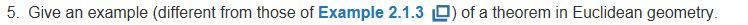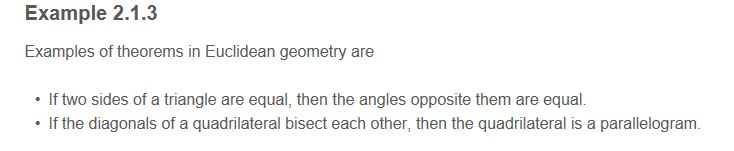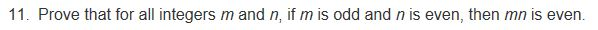• Section 2.2, page 81, problems 9, 14.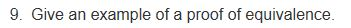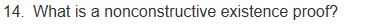• Section 2.3, page 88, ONLY problem 6. See example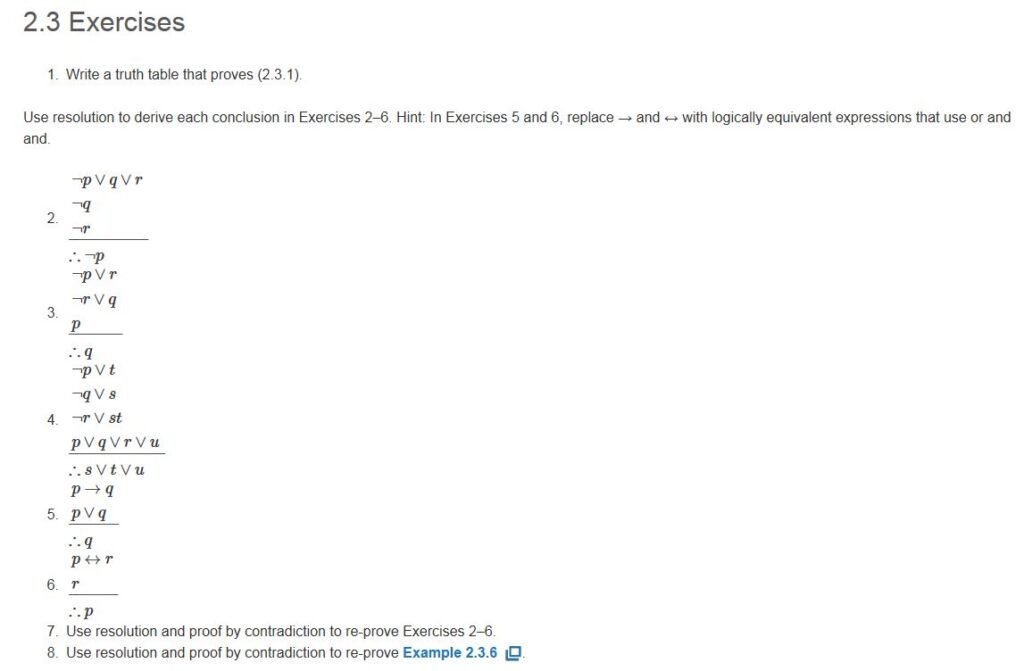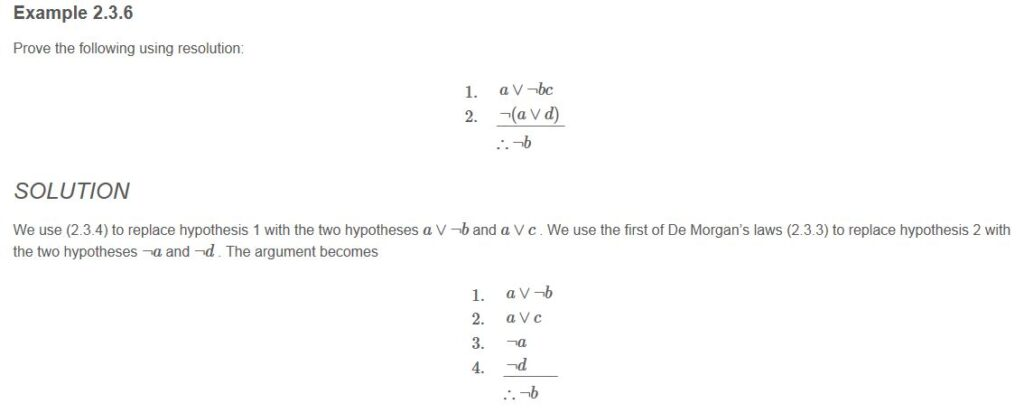• Section 2.4, page 96, problems 3, 9. ONLY 3 and 9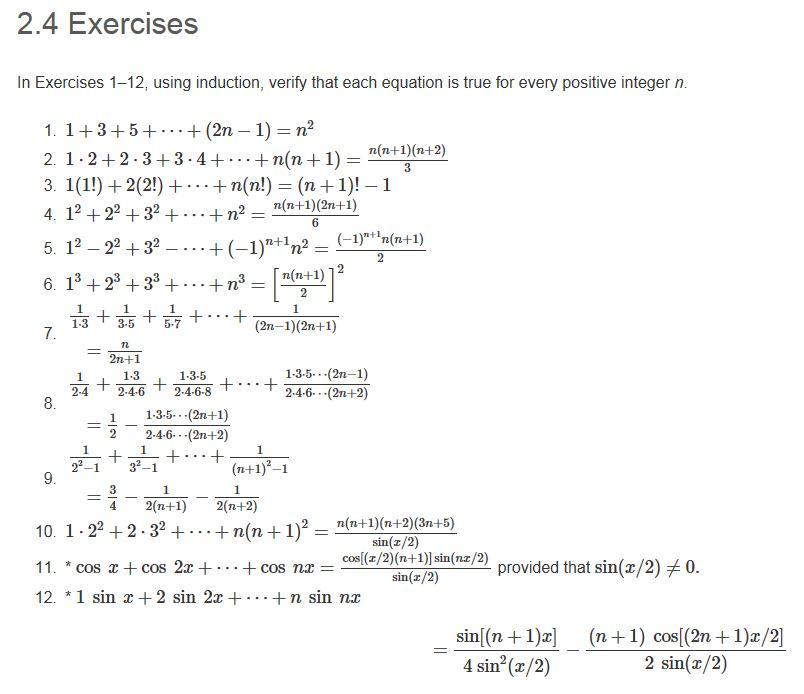Calculate: Questions 1 and 2

1. Given that an IP address in IPv4 is a 32-bit string, how many different addresses can be encoded? Show your calculation. Hint: Use the multiplication principle.
1. How many different Web sites could be encoded using IPv6? Show your calculation.

Math U5 Lesson 1

• Section 4.1, page 176, problem 5.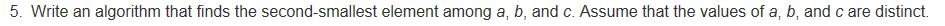• Section 4.2, page 183, problems 5, 9. Algorithms for 5 and 9 below the problems.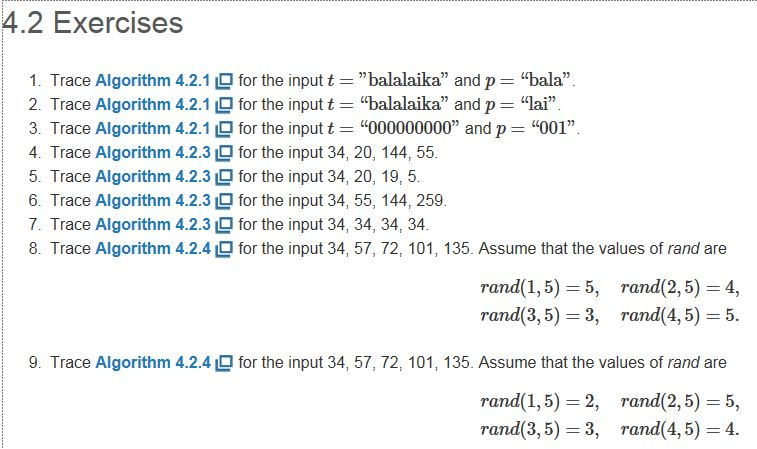• Section 4.3, page 198, problems 2, 15, 19, 21. ONLY 2, 15, 19, 21.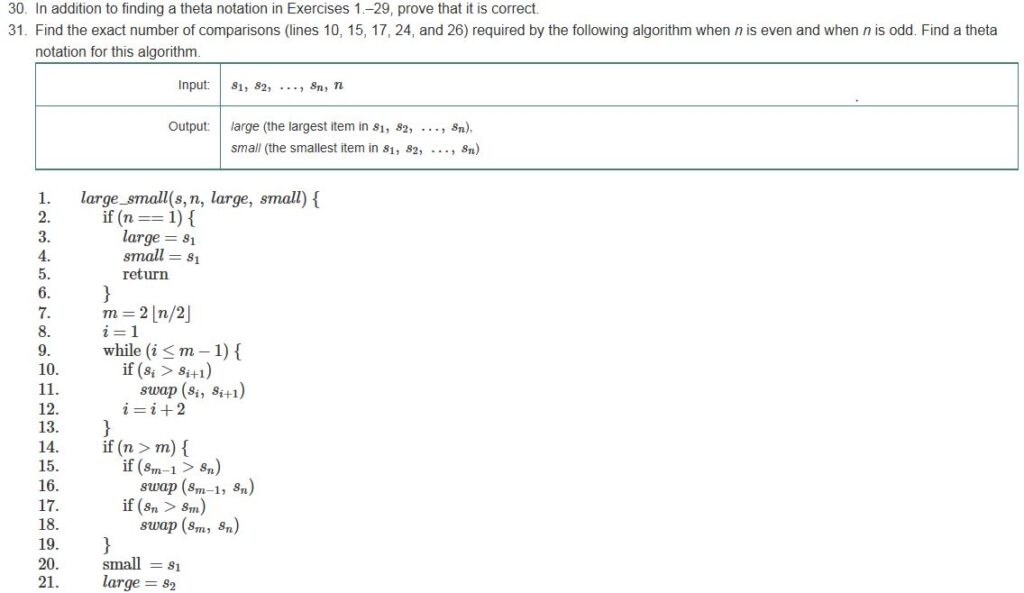• Appendix C, page 625, problems 2, 8. Examples c.7 and c.9 below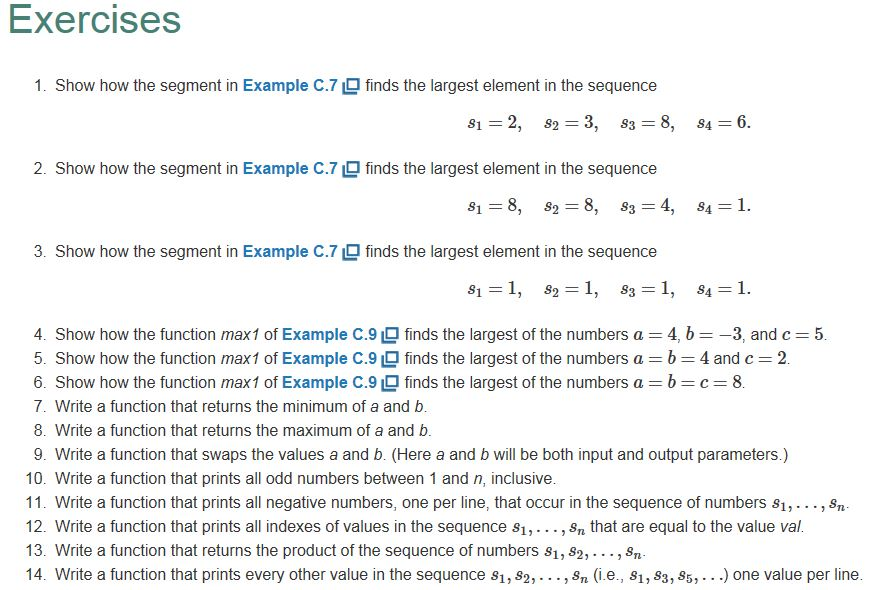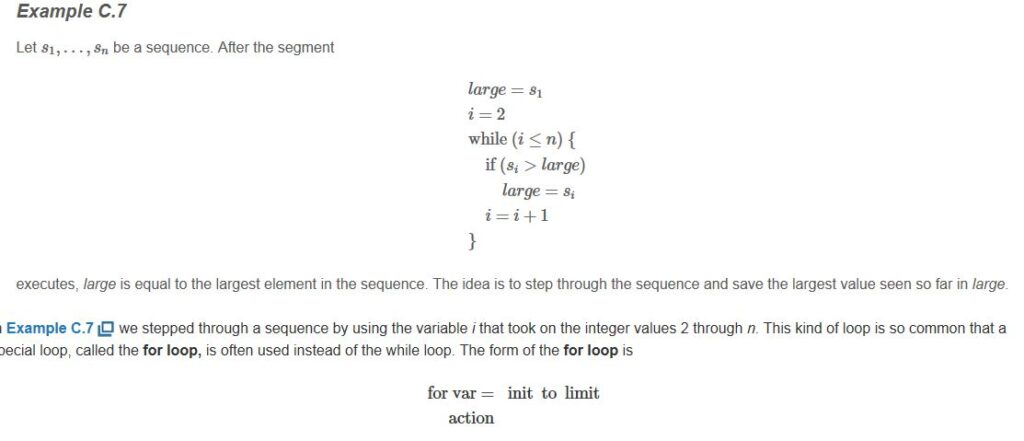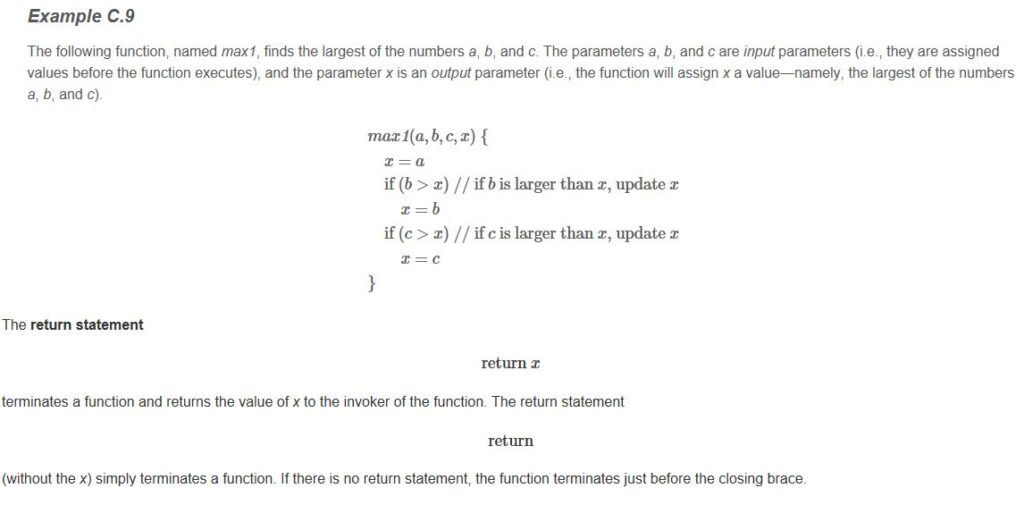Algorithms and Time Complexity Questions 1 and 2

1. What is time complexity?
2. What is space complexity?

1. Provide an example of an algorithm for each worst-case run times:
1. O( n).
2. O( nk). Note that this is called polynomial-time, where k is any number greater than 1.
3. NP-time.

Hint: Quick sort is an algorithm that runs in O( nlog n) time.

Math U4 Lesson 1

• Section 2.1, page 70, problems 5, 11.

Ans no. 5 :

Example of theorem in Euclidean geometry is:-

If two parallel lines are cut by a transversal, then both pairs of alternate interior angles are congruent.”

Ans no. 11 :

Lets suppose an odd number be m = 2a+1 and one even number be n = 2b . (where a and b are integers)

Now, their product i.e. m*n = (2a +1)*(2b)

= 4ab + 2b

= 2(2ab + b) [an even number form]

Hence, it is proved that mn is an even number.

• Section 2.2, page 81, problems 9, 14.

Ans no. 9 :

When a relation follows all three criteria i.e. reflexive , symmetric and transitive.

It is called as an equivalence relation.

Example: A relation R “line a is parallel to line b” is an equivalence relation.

Proof:

For Reflexive-

Since a line is parallel to itself , hence the given relation is reflexive.

For Symmetric-

If aRb exists there must be existing bRa. If line a is parallel to line b then this means line b is also parallel to line a. Hence this relation is symmetric.

For Transitive-

If aRb and bRc then aRc. If line a is parallel to line b and line b is parallel to line c then it is very clear that line a will be parallel to line c also.

Hence, it is transitive also.

Since, given relation R is all reflexive , symmetric and transitive. Hence relation R is an equivalence relation.

Ans no. 14 :

proof which indirectly shows a mathematical object exists without providing a specific example or algorithm for producing an example is called as nonconstructive existence proof.

• Section 2.3, page 88, ONLY problem 6. See example

Ans no. 6:

1. pr

2. r

p

Solution:

We use equivalent expression of pr as (¬p r) ^ (¬r p).

1. ¬p r

2. ¬r p

3. r

p

Section 2.4, page 96, problems 3, 9. ONLY 3 and 9

Ans no. 3:

1(1!) + 2(2!) + ……..+ n(n!) = (n+1)! – 1

Let, f(n) = 1(1!)+2(2!)+….+n(n!) = (n+1)! – 1

t(n) = n(n!),

Let us assume that the formula is true for n=k,

that is,

1(1!)+2(2!)+…..+k(k!) = (k+1)! – 1……… eq (1)

Now, for n=k+1, the formula will be,

1(1!)+2(2!)+…..+k+1(k+1!) = (k+2)! -1..….eq(2)

Now, f(k+1) should be equal to f(k)+t(k+1) for the statement to be true for every positive number.

f(k)+t(k+1)=1(1!)+2(2!)+….+k(k!)+(k+1)((k+1)!)=(k+1)! – 1 + (k+1)((k+1)!) =(k+1)! + (k+1)((k+1)!) – 1

Taking (k+1) common,

=(1+k+1)(k+1)! – 1 =(k+2)(k+1)! – 1 =(k+2)! – 1

Hence proved.

Now, the statement should be true for n=1

f(1)=1(1!)=1 = (1+1)! – 1= 2! – 1= 2-1 =1

Hence proved.

We saw the statement fulfils both the conditions of mathematical indection, hence, it is true for all positive integers.

Ans no. 9:

1/(22-1) + 1/(32-1) + ……… + 1/((n+1)2-1) = ¾ – 1/2(n+1) – 1/2(n+2)

Let, f(n) = 1/(22-1)+1/(32-1)+………+ 1/((n+1)2-1) = ¾ – 1/2(n+1) – 1/2(n+2)

t(n) = 1/((n+1)2-1),

Let us assume that the formula is true for n=k,

that is,

1/(22-1)+1/(32-1)+………+1/((k+1)2-1) = ¾-1/2(k+1) – 1/2(k+2)……..eq(1)

Now, for n=k+1, the formula will be,

1/(22-1)+1/(32-1)+………+ 1/((k+1+1)2-1) = ¾-1/2(k+2) – 1/2(k+3)..…..eq(2)

Now, f(k+1) should be equal to f(k)+t(k+1) for the statement to be true for every positive number.

f(k)+t(k+1)=1/(22-1)+1/(32-1)+……..+1/((k+1)2-1)+ ((k+2)2-1) =¾-½(k+1)-½(k+2)+1/((k+2)2-1) =¾-½(k+2)-1/(2k+2)+ 1/(k2+k+3k+3) =¾-½(k+2)+(-k2-4k-3+2k+2)/2(k+1)(k+1)(k+3) =¾-½(k+2)-(k2+2k+1)/2(k+1)2(k+3) =¾-½(k+2)-½(k+3)=f(k+1)

Hence proved.

Now, the statement should be true for n=1

f(1)=1/(22-1)=1/3= ¾-½(1+1)-½(1+2) = ¾-¼-⅙ = ⅓

Hence proved.

We saw the statement fulfils both the conditions of mathematical indection, hence, it is true for all positive integers.

Calculate: Questions 1 and 2

Ans no. 1: Given that an IP address in IPv4 is a 32-bit string, how many different addresses can be encoded? Show your calculation. Hint: Use the multiplication principle.

We know each bits consist of ones or zeros and given IPv4 is a 32-bit string, so the number of different addresses that can be encoded are C(2,32)=232=4,294,967,296

Ans no. 2 : How many different Web sites could be encoded using IPv6? Show your calculation.

The number of different Web sites that can be encoded using IPv6 are 2128=340,282,366,920,938,463,463,374,607,431,768,211,456.

Math U5 Lesson 1

• Section 4.1, page 176, problem 5

5.)

Start

Initialize variable a, b, c, lar, seclar

If a>b

If a>c

lar=a

else

seclar=a

if b>c

if(b>a)

lar=a

else

seclar=b

if(c>a)

if(c>b)

lar=c

else

seclar=c

Stop

• Section 4.2, page 183, problems 5, 9. Algorithms for 5 and 9 below the problems.

5.)

s=(34,20,19,5), n=4

Start

Loop begins

Initialize i=2

val=si=s2=20

j=i-1=2-1=1, sj=34

Loop begins

j=1 and val<sj

sj+1=s1+1=s2=sj=s1=34

j=j-1=1-1=0

j<1

Loop ends

sj+1=s1=val=20 //s=(20, 34,19,5)

Increment i to 3

val=si=s3=19

j=i-1=3-1=2, sj=s2=34

Loop begins

j=2 and val<sj

sj+1=s2+1=s3=sj=s2=34

j=j-1=2-1=1

j=1 and val<sj

sj+1=s1+1=s2=sj=s1=20

j=j-1=1-1=0

j<1

Loop ends

sj+1=s1=val=19 //s=(19,20,34,5)

Increment i to 4

val=si=s4=5

j=i-1=4-1=3, sj=s3=34

Loop begins

j=3 and val<sj

sj+1=s3+1=s4=sj=s3=34

j=j-1=3-1=2

j>1 and val<sj

sj+1=s2+1=s3=sj=s2=20

j=j-1=2-1=1

j=1 and val<sj

sj+1=s1+1=s2=sj=s1=19

j=j-1=1-1=0

j<1

Loop ends

sj+1=s1=val=5 //s=(5,19,20,34)

Loop ends

Stop

9.)

s=(34,57,72,101,135), n=5

rand(1,5)=2

rand(2,5)=5

rand(3,5)=3

rand(4,5)=4

Start

Loop begins

Initialize i=1

swap(a1, arand(1,5))

swap(34,57) //s=(57,34,72,101,135)

Increment i to 2

swap(a2, arand(2,5))

swap(34,135) //s=(57,135,72,101,34)

Increment i to 3

swap(a3, arand(3,5))

swap(72,72) //s=(57,135,72,101,34)

Increment i to 4

swap(a4, arand(4,5))

swap(101,101) //s=(57,135,72,101,34)

Loop ends

• Appendix C, page 625, problems 2, 8. Examples c.7 and c.9 below

2.)

s1=8, s2=8, s3=4, s4=1, n=4

Start

Initialize large=s1=8

Initialize i=2

Loop begins

i<4

si=s2=large

i=i+1=2+1=3

i<4

si=s3<large

i=i+1=3+1=4

i=4

si=s4<large

i=i+1=4+1=5

i>4

Loop ends

Stop

large = largest number = 8

8.)

int max(int a, int b)

{

If(a>b)

return a;

return b //default output will be b.

}

Algorithms and Time Complexity Questions 1 and 2

1. What is time complexity?

Time complexity of an algorithm quantifies the amount of time taken by an algorithm to run as a function of the length of the input.

1. What is space Complexity?

Space complexity of an algorithm quantifies the amount of space or memory taken by an algorithm to run as a function of the length of the input.

1. Provide an example of an algorithm for each worst-case run times:

1. O( n).

Operations on Binary search tree, etc

1. O( nk). Note that this is called polynomial-time, where k is any number greater than 1.

Minimum spanning tree

1. NP-time.

Travelling salesperson problem

Hint: Quick sort is an algorithm that runs in O( nlog n) time.

Looking for best Mathematics Assignment Help. Whatsapp us at +16469488918 or chat with our chat representative showing on lower right corner or order from here. You can also take help from our Live Assignment helper for any exam or live assignment related assistance.